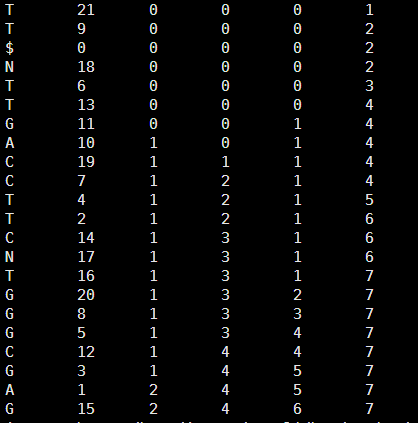# Bowtie算法第六讲-tally法对bwt索引进行搜索

perl rotation_one_by_one.pl atgtgtcgtagctcgtnncgt

\$ATGTGTCGTAGCTCGTNNCGT 21

AGCTCGTNNCGT\$ATGTGTCGT 9

ATGTGTCGTAGCTCGTNNCGT\$ 0

CGT\$ATGTGTCGTAGCTCGTNN 18

CGTAGCTCGTNNCGT\$ATGTGT 6

CGTNNCGT\$ATGTGTCGTAGCT 13

CTCGTNNCGT\$ATGTGTCGTAG 11

GCTCGTNNCGT\$ATGTGTCGTA 10

GT\$ATGTGTCGTAGCTCGTNNC 19

GTAGCTCGTNNCGT\$ATGTGTC 7

GTCGTAGCTCGTNNCGT\$ATGT 4

GTGTCGTAGCTCGTNNCGT\$AT 2

GTNNCGT\$ATGTGTCGTAGCTC 14

NCGT\$ATGTGTCGTAGCTCGTN 17

NNCGT\$ATGTGTCGTAGCTCGT 16

T\$ATGTGTCGTAGCTCGTNNCG 20

TAGCTCGTNNCGT\$ATGTGTCG 8

TCGTAGCTCGTNNCGT\$ATGTG 5

TCGTNNCGT\$ATGTGTCGTAGC 12

TGTCGTAGCTCGTNNCGT\$ATG 3

TGTGTCGTAGCTCGTNNCGT\$A 1

TNNCGT\$ATGTGTCGTAGCTCG 15

T 21

T 9

\$ 0

N 18

T 6

T 13

G 11

A 10

C 19

C 7

T 4

T 2

C 14

N 17

T 16

G 20

G 8

G 5

C 12

G 3

A 1

G 15perl search_char.pl    GTGTCG   tm.tally

your last char is G

start is 7 ; and end is 13

now it is number 5 and the char is C

start is 3 ; and end is 6

now it is number 4 and the char is T

start is 17 ; and end is 19

now it is number 3 and the char is G

start is 10 ; and end is 11

now it is number 2 and the char is T

start is 19 ; and end is 20

now it is number 1 and the char is G

start is 11 ; and end is 12

It is just one perfect match !

The index is 2

perl search_char.pl  GT  tm.tally

your last char is T

start is 15 ; and end is 22

now it is number 1 and the char is G

start is 8 ; and end is 13

we find more than one perfect match!!!

8 13

One of the index is 11

One of the index is 10

One of the index is 19

One of the index is 7

One of the index is 4

One of the index is 2

One of the index is 14

CTCGTNNCGT\$ATGTGTCGTAG 11

GCTCGTNNCGT\$ATGTGTCGTA 10

GT\$ATGTGTCGTAGCTCGTNNC 19

GTAGCTCGTNNCGT\$ATGTGTC 7

GTCGTAGCTCGTNNCGT\$ATGT 4

GTGTCGTAGCTCGTNNCGT\$AT 2

GTNNCGT\$ATGTGTCGTAGCTC 14

perl search_char.pl ACATGTGT tm.tally

your last char is T

start is 15 ; and end is 22

now it is number 7 and the char is G

start is 8 ; and end is 13

now it is number 6 and the char is T

start is 19 ; and end is 21

now it is number 5 and the char is G

start is 11 ; and end is 12

now it is number 4 and the char is T

start is 20 ; and end is 21

now it is number 3 and the char is A

start is 2 ; and end is 3

now it is number 2 and the char is C

start is 3 ; and end is 3

we can just find the last 6 char ,and it is ATGTGT

[perl]

\$a=\$ARGV;

\$a=uc \$a;

open FH,"<\$ARGV";

while(<FH>){

chomp;

@F=split;

\$hash_count_atcg{\$F}++;

\$hash{\$.}=\$_;

# the first line is \$ and the last char and the last index !

}

\$all_a=\$hash_count_atcg{'A'};

\$all_c=\$hash_count_atcg{'C'};

\$all_g=\$hash_count_atcg{'G'};

\$all_n=\$hash_count_atcg{'N'};

\$all_t=\$hash_count_atcg{'T'};

#print "\$all_a\t\$all_c\t\$all_g\t\$all_t\n";

\$len_a=length \$a;

\$end_a=\$len_a-1;

#print "your query is \$a\n";

#print "and the length of your query is \$len_a \n";

\$after=substr(\$a,\$end_a,1);

#we fill search your query from the last char !

if (\$after eq 'A') {

\$start=2;

\$end=\$all_a+1;

}

elsif (\$after eq 'C') {

\$start=\$all_a+1;

\$end=\$all_a+\$all_c+1;

}

elsif (\$after eq 'G') {

\$start=\$all_a+\$all_c+1;

\$end=\$all_a+\$all_c+\$all_g+1;

}

elsif (\$after eq 'T'){

\$start=\$all_a+\$all_c+\$all_g+\$all_n+1;

\$end=\$all_a+\$all_c+\$all_g+\$all_t+\$all_n+1;

}

else {die "error !!! we just need A T C G !!!\n"}

print "your last char is \$after\n ";

print "start is \$start ; and end is \$end \n";

foreach (reverse (1..\$end_a)){

\$after=substr(\$a,\$_,1);

\$before=substr(\$a,\$_-1,1);

(\$start,\$end)=&find_level(\$after,\$before,\$start,\$end);

print "now it is number \$_ and the char is \$before \n ";

print "start is \$start ; and end is \$end \n";

if (\$_  > 1 && \$start == \$end) {

\$find_char=substr(\$a,\$_);

\$find_len=length \$find_char;

print "we can just find the last \$find_len char ,and it is \$find_char \n";

#return "miss";

last;

}

if (\$_ == 1) {

if ((\$end-\$start)==1) {

print "It is just one perfect match ! \n";

my @F_start=split/\s+/,\$hash{\$end};

print "The index is \$F_start\n";

#return \$F_start;

last;

}

else {

print "we find more than one perfect match!!!\n";

print "\$start\t\$end\n";

foreach  ((\$start-1)..\$end) {

my @F_start=split/\s+/,\$hash{\$_};

print "One of the index is \$F_start\n";

}

#return "multiple";

last;

}

}

}

sub find_level{

my(\$after,\$before,\$start,\$end)=@_;

my @F_start=split/\s+/,\$hash{\$start};

my @F_end=split/\s+/,\$hash{\$end};

if (\$before eq 'A') {

return (\$F_start+1,\$F_end+1);

}

elsif (\$before eq 'C') {

return (\$all_a+\$F_start+1,\$all_a+\$F_end+1);

}

elsif (\$before eq 'G') {

return (\$all_a+\$all_c+1+\$F_start,\$all_a+\$all_c+1+\$F_end);

}

elsif (\$before eq 'T') {

return (\$all_a+\$all_c+\$all_g+\$all_n+1+\$F_start,\$all_a+\$all_c+\$all_g+1+\$all_n+\$F_end);

}

else {die "error !!! we just need A T C G !!!\n"}

}

[/perl]